# Circles-Sample Questions

Class X Math
Sample Paper for Facts that Matter
1.   In the figure, PA is a tangent from an external point P to a circle with centre O. If ∠POB = 115° then find ∠APO.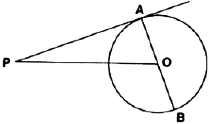2.   In the following figure, PA and PB are tangents drawn from a point P to the circle with centre O. If ∠APB = 60°, then what is ∠AOB?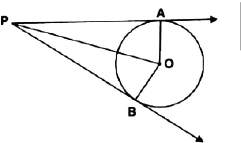3.   In the figure, CP and CQ are tangents to a circle with centre O. ARB is another tangent touching the circle at R. If QC = 11 cm, BC = 7 cm then find, teh length of BR.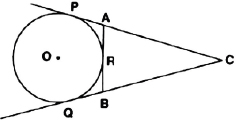4.   In the figure, ��ABC is circumscribing a circle. Find the length of BC.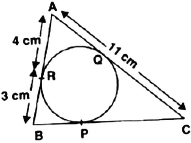5.   In the figure, if ∠ATO = 40°, find ∠AOB.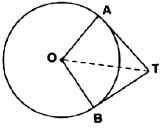6.   From a point P, the length of the tangent to a circle is 15 cm and distance of P from the centre of the circle is 17 cm, then what is the radius of the circle?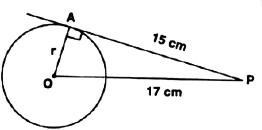7.   The two tangents from an external point P to a circle with centre O are PA and PB. If ∠APB = 70°, then what is the value of ∠AOB?
8.   Two tangents TP and TQ are drawn to a circle with centre O from an external point T. Prove that ∠PTQ = 2 ∠OPQ.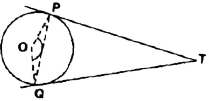9.   A circle is touching the side BC of a ��ABC at P and touchign AB and AC produced at Q and R.
Prove that: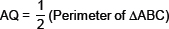10.   In two concentric circle, a chord of the larger circle touches the smaller circle. If the length of this chord is 8 cm and the diameter of the smaller circle is 6 cm, then find the diameter of the larger circle.
11.   In the following figure, PA and PB are two tangents drawn to a circle with centre O, from an external point P such that PA = 5 cm and ∠APB = 60°. Find the length of chord AB.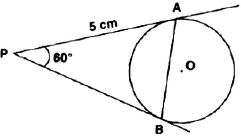12.   In the following figure, AB is a chord of length 9.6 cm of a circle with centre O and radius 6 cm.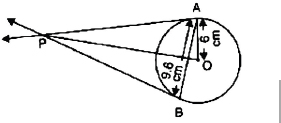The tangents at A and B intersect at P. Find the length PA.
13.   Two tangents PA and PB are drawn to a circle with centre O from an external point P. Prove that
∠APB = 2∠OAB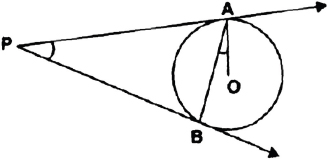14.   ABC is an isosceles triangle, in which AB = AC, circumscribed about a circle. Show that BC is bisected at the point of contact.
15.   Prove that the angle between the two tangents to a circle drawn from an external point is supplementary to the angle subtended by the line segment joining the points of contact at the centre.
Or
Two tangents PA and PB are drawn from an external point P to a circle with centre O. Prove that AOBP is a cyclic quadrilateral.